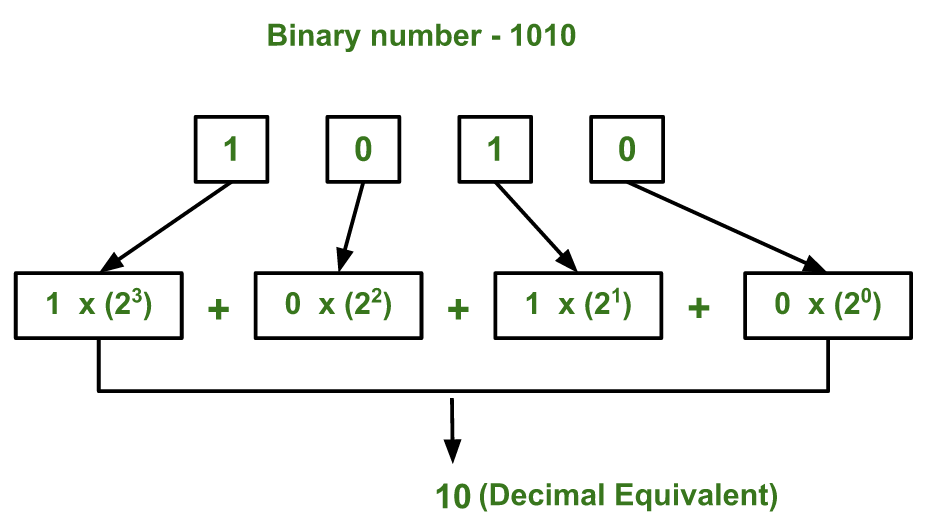# Recursive Program for Binary to Decimal

Given a binary number as string, find its decimal equivalent.

Examples:

```Input :  binary = "101"
Output :   5

Input :  binary = "1111"
Output :   15
```

## Recommended: Please try your approach on {IDE} first, before moving on to the solution.

We have discussed iterative solution to convert Binary to Decimal.

The idea is simple, we add current term and recur for remaining terms.## C++

 `// Recursive CPP program to convert binary  ` `// decimal ` `#include ` `using` `namespace` `std; ` ` `  `int` `toDecimal(string binary, ``int` `i=0) ` `{ ` `    ``// If we reached last character ` `    ``int` `n = binary.length(); ` `    ``if` `(i == n-1) ` `      ``return` `binary[i] - ``'0'``; ` `     `  `    ``// Add current tern and recur for ` `    ``// remaining terms ` `    ``return` `((binary[i] - ``'0'``) << (n-i-1))  + ` `            ``toDecimal(binary, i+1); ` `} ` ` `  `// Driver code ` `int` `main() ` `{ ` `    ``string binary = ``"1010"``; ` `    ``cout << toDecimal(binary) << endl; ` `    ``return` `0; ` `} `

## Java

 `// Recursive Java program to convert binary  ` `// decimal ` ` `  ` `  `class` `GFG ` `{ ` `    ``static` `int` `toDecimal(String binary,``int` `i) ` `    ``{ ` `        ``// If we reached last character ` `        ``int` `n = binary.length(); ` `        ``if` `(i == n-``1``) ` `        ``return` `binary.charAt(i) - ``'0'``; ` `         `  `        ``// Add current tern and recur for ` `        ``// remaining terms ` `        ``return` `((binary.charAt(i) - ``'0'``) << (n-i-``1``)) + ` `                ``toDecimal(binary, i+``1``); ` `    ``} ` `     `  `    ``// Driver code ` `    ``public` `static` `void` `main(String []args) ` `    ``{ ` `        ``String binary = ``"1010"``; ` `        ``int` `i=``0``; ` `        ``System.out.println(toDecimal(binary,i)); ` `         `  `    ``} ` ` `  `} ` ` `  `// This code is contributed  ` `// by ihritik ( Hritik Raj) `

## Python3

 `# Recursive Python3 program to convert  ` `# binary decimal  ` ` `  `def` `toDecimal(binary, i ``=` `0``): ` ` `  `    ``# If we reached last character  ` `    ``n ``=` `len``(binary)  ` `    ``if` `(i ``=``=` `n ``-` `1``) : ` `        ``return` `int``(binary[i]) ``-` `0` `     `  `    ``# Add current tern and recur for  ` `    ``# remaining terms  ` `    ``return` `(((``int``(binary[i]) ``-` `0``) << (n ``-` `i ``-` `1``)) ``+`  `                        ``toDecimal(binary, i ``+` `1``)) ` ` `  `# Driver code  ` `if` `__name__ ``=``=` `"__main__"` `: ` `     `  `    ``binary ``=` `"1010"` `    ``print``(toDecimal(binary)) ` ` `  `# This code is contributed by Ryuga `

## C#

 `// Recursive C# program to convert binary  ` `// decimal ` ` `  ` `  `using` `System; ` `class` `GFG ` `{ ` `    ``static` `int` `toDecimal(``string` `binary, ``int` `i=0) ` `    ``{ ` `        ``// If we reached last character ` `        ``int` `n = binary.Length; ` `        ``if` `(i == n-1) ` `        ``return` `binary[i] - ``'0'``; ` `         `  `        ``// Add current tern and recur for ` `        ``// remaining terms ` `        ``return` `((binary[i] - ``'0'``) << (n-i-1)) + ` `                ``toDecimal(binary, i+1); ` `    ``} ` `     `  `    ``// Driver code ` `    ``public` `static` `void` `Main() ` `    ``{ ` `        ``string` `binary = ``"1010"``; ` `        ``Console.WriteLine(toDecimal(binary)); ` `         `  `    ``} ` ` `  `} ` ` `  `// This code is contributed  ` `// by ihritik ( Hritik Raj) `

## PHP

 ` `

Output:

```10
```

My Personal Notes arrow_drop_upCheck out this Author's contributed articles.

If you like GeeksforGeeks and would like to contribute, you can also write an article using contribute.geeksforgeeks.org or mail your article to contribute@geeksforgeeks.org. See your article appearing on the GeeksforGeeks main page and help other Geeks.

Please Improve this article if you find anything incorrect by clicking on the "Improve Article" button below.

Article Tags :
Practice Tags :

Be the First to upvote.

Please write to us at contribute@geeksforgeeks.org to report any issue with the above content.